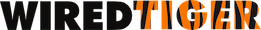Version 2.8.0
Join cursors in Java

Join cursors provide a way to iterate over a subset of a table, where the subset is specified by relationships with reference cursors.

A join cursor is created with Session.open_cursor using a `"join:table:<name>"` URI prefix. Then reference cursors are positioned to keys on indices and joined to the join cursor using Session.join calls. The result is a join cursor that can be iterated to satisfy the join equation.

Here is an example using join cursors:

/* Open cursors needed by the join. */
join_cursor = session.open_cursor(
"join:table:poptable", null, null);
cursor = session.open_cursor(
"index:poptable:country", null, null);
cursor2 = session.open_cursor(
"index:poptable:immutable_year", null, null);
/* select values WHERE country == "AU" AND year > 1900 */
cursor.putKeyString("AU");
ret = cursor.search();
session.join(join_cursor, cursor, "compare=eq,count=10");
cursor2.putKeyShort((short)1900);
ret = cursor2.search();
session.join(join_cursor, cursor2,
"compare=gt,count=10,strategy=bloom");
/* List the values that are joined */
while ((ret = join_cursor.next()) == 0) {
recno = join_cursor.getKeyRecord();
country = join_cursor.getValueString();
year = join_cursor.getValueShort();
population = join_cursor.getValueLong();
System.out.print("ID " + recno);
System.out.println( ": country " + country + ", year " + year +
", population " + population);
}

Joins support various comparison operators: `"eq"`, `"gt"`, `"ge"`, `"lt"`, `"le"`. Ranges with lower and upper bounds can also be specified, by joining two cursors on the same index, for example, one with `"compare=ge"` and another `"compare=lt"`. In addition to joining indices, the main table can be joined so that a range of primary keys can be specified.

All the joins should be done on the join cursor before Cursor.next is called. Calling Cursor.next on a join cursor for the first time populates any bloom filters and performs other initialization. The join cursor's key is the primary key (the key for the main table), and its value is the entire set of values of the main table. A join cursor can be created with a projection by appending `"(col1,col2,...)"` to the URI if a different set of values is needed.